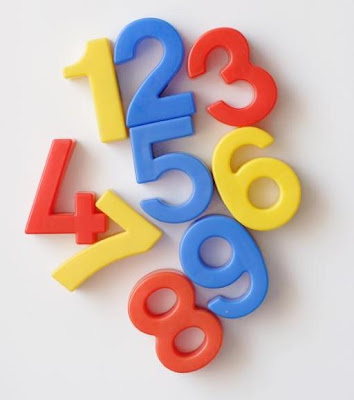Another Math Trick !

posted by Di-G onMultiply 37,037 by any single number (1-9),

then multiply that number by 3.

Every digit in the answer will be the same as that first single number.

For example:
37,037*5=185,185.
185,185*3=555,555.